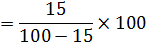# Percentage Tricks With Examples Part-2

1.  If the price of any foodstuff is decreased by 'a%' then the consumption of it will be increased by% so that the expense of this item is remaining same.

Example
The price of tea is decreased by 15%. How much % consumption of sugar is increased so that the expenditure of sugar is same?=17.65%

2. If a number is increase/ decrease by X% and then increase/ decrease by Y% soExample
If a number is increased by 10% and then decreased by 5%. Find the total % change in that number.=10-5-.5
=4.5%

## Some more important Examples:

#### Example 1.

20% of a number is 60 less than three-fifth of that number. Find that number.
Solution: x×3/5 - x×20% = 60
x×3/5 - x×1/5 =60
x×2/5=60
x=150

#### Example 2.

The length of rectangular is increased by 8% while the width is increased by 10%. What is the % change in this area?
Solution:=18.8%

#### Example 3.

Mohan gave 40% of his money to his wife and gave 20% of the remaining money to his daughter and he donated half of the amount he left and now he has only 1500 rs. How much money did Mohan have initially?
Solution:
Let the total money= xX×60%×80%×50%=1500
X=6250 rs.

#### Example 4.

In the three sided election A gets 12% of the total votes and remain on 3rd position, B gets 46% of votes and he wins the election by 1200 votes, C remains on 2nd position. Find the total votes.
Solution:12% 46% 42%
46%-42%=4%
4%=1200
100%=30000

#### Example 5.

Two numbers are respectively 20% and 35% more than 3rd number. 2nd number is how much % more than 1st.
Solution:
3rd number= 100, 1st number= 120, 2nd number= 135=15/120×100
=12.5%

#### Example 6.

In an examination 33% of total marks are passing marks. 1 candidate gets 150 marks and failed by 48 marks. Find the total marks.
Solution:
Total passing marks= 150+45 =198
33% = 198
100% = 600
Total marks= 600

#### Example 7.

In an examination 35% of the total marks are passing marks. 1 candidate got 120 marks and failed by 5% marks. Find the passing marks.
Solution:
Candidate got 35%- 5%
30% = 120
100% = 400

#### Example 8.

In an examination 1 candidate got 30% marks and failed by 40 marks another candidate secure 40% marks and got 40 marks more than the passing marks. Find the passing marks.
Solution:
X×30%+40= X×40%-40
X×40%-X×30% = 40+40
X×10%=80
X=800
Passing marks = 800×30% + 40
=240+40
=280